# Gnuplotting

Create scientific plots using gnuplot

September 8th, 2011 | 3 Comments

In the last entry about attractive plots we introduced the usage of gray borders and tics in order to emphasize the content of the figure.
If we want to do the same in the `epslatex` terminal, we had to add the color of the text directly with some LaTeX code:

```set terminal epslatex size 10.4cm,6.35cm color colortext standalone \
set format '\color{tics}\$%g\$'
```

The problem is that the output looks not the way it should be, but like the one in Fig. 1.
It appears that all the letters we have written in the format string are used for the calculation of the size of the tics.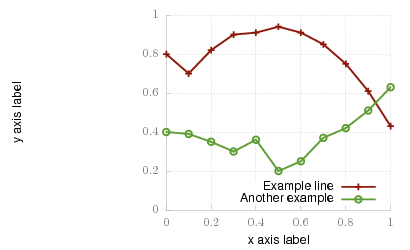Fig. 1 Colored tics plotted with Gnuplot version <4.4.3 (code to produce this figure)

An easy solution to that problem is to use Gnuplot 4.4.3, because the size calculation has been updated. Fig. 2 shows the result if we use Gnuplot 4.4.3 to create the figure. But still there is some room for improvements.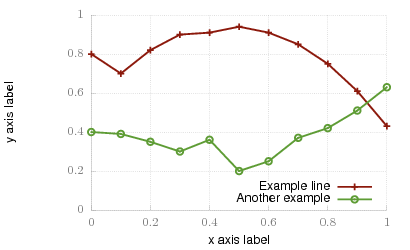Fig. 2 Colored tics plotted with Gnuplot 4.4.3 (code to produce this figure)

The length of the color name has still an influence on the calculated tics size. Hence we use only one letter for the color definition:

```set terminal epslatex size 10.4cm,6.35cm color colortext standalone \
set format '\color{t}\$%g\$'
```

The final result is shown in Fig. 3.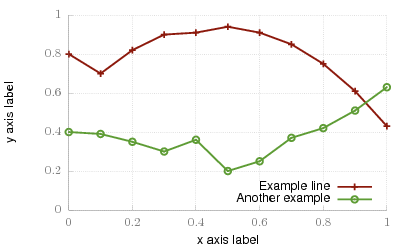Fig. 3 Colored tics plotted with Gnuplot 4.4.3 (code to produce this figure)

August 11th, 2011 | 8 Comments

As you surely have noticed I don’t use the default colors and line styles from Gnuplot, but define them myself. The simple reason is that the default colors are not optimized to be very pleasant, but are simply primary colors. I just stumbled over an blog entry of Brighten Godfrey, which deals with some thoughts on beautiful plots.
He suggest to create scientific plots like the way he created his figure which I have reproduced more or less accurate in Fig. 1.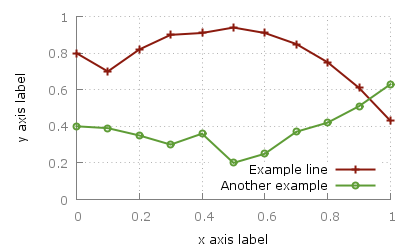Fig. 1 Nice plot with the pngcairo terminal (code to produce this figure, data)

In Fig. 2 the default output of the `pngcairo` terminal is shown. I think the difference is quiet obvious.Fig. 2 Default output of the pngcairo terminal (code to produce this figure, data)

In the following I will have a look at the things we have to do to reach Fig. 1 and why we should do this:

1) change the default colors to more pleasant ones and make the lines a little bit thicker

```set style line 1 lc rgb '#8b1a0e' pt 1 ps 1 lt 1 lw 2 # --- red
set style line 2 lc rgb '#5e9c36' pt 6 ps 1 lt 1 lw 2 # --- green
```

2) put the border more to the background by applying it only on the left and bottom part and put it and the tics in gray

```set style line 11 lc rgb '#808080' lt 1
set border 3 back ls 11
set tics nomirror
```

3) add a slight grid to make it easier to follow the exact position of the curves

```set style line 12 lc rgb '#808080' lt 0 lw 1
set grid back ls 12
```

The last thing I would like to mention is the problem, that the output of the `svg` terminal is slightly different from the `pngcairo` terminal. Especially the dashed line of the grid is not created in the right way, even though the `dashed` option is used for the terminal. This and a solution to convert the lines to dashed versions is also mentioned in the plotting the world entry.

Fig. 1 Nice plot with the svg terminal (code to produce this figure, data)

March 15th, 2011 | No Comments

As you all know Gnuplot is able to add arrows or labels to your plot. But it can even add more complicated objects, by using the `object` command. You can choose if you want to add a rectangle, ellipse, circle, or polygon. Here we want to add a loudspeaker to our plot, hence we choose the polygon.

Fig. 1 A loudspeaker drawn with the object command (code to produce this figure)

For a polygon you have to specify the points the polygon should combine. The following points will result in a shape of the desired loudspeaker.

```a = 1.0/3
set object 1 polygon from \
-1, 1 to \
0, 1 to \
0, a to \
1, 1 to \
1,-1 to \
0,-a to \
0,-1 to \
-1,-1 to \
-1, 1
```

After this we do some fine tuning on the appearance of the object and remove all borders and tics from the plot.

```unset border
unset tics
set object 1 fc rgb '#000000' fillstyle solid lw 0
```

In order to draw the loudspeaker we need a plot command. For this we use the parametric mode to draw parts of circles with different radii. Here I will only present the used functions, for an explanation of the parametric mode have a look at the Understand parametric mode entry.

```set trange [-pi/6:pi/6]
fx(t,r) = r*cos(t)
fy(t,r) = r*sin(t)
```

October 27th, 2010 | 11 Comments

If we have more than one graph that should be displayed in a figure, the `multiplot` command is the one to use in Gnuplot. But as we will see this is not a trivial task.
Let us consider we have four different functions that should be presented in the same figure as shown in Fig. 1.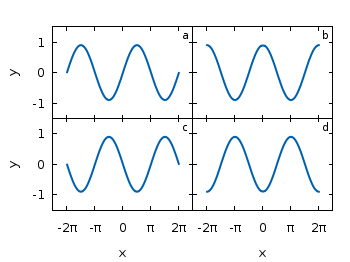Fig. 1 A multiplot with reduced axes labeling and nicely arranged graphs (code to produce this figure)

The functions are given by:

```# Functions (1/0 means not defined)
a = 0.9
f(x) = abs(x)<2*pi ? a*sin(x)           : 1/0
g(x) = abs(x)<2*pi ? a*sin(x+pi/2)      : 1/0
h(x) = abs(x)<2*pi ? a*sin(x+pi)        : 1/0
k(x) = abs(x)<2*pi ? a*sin(x+3.0/2*pi)  : 1/0
```

For an explanation of the used syntax to declare the functions have a look at the Defining piecewise functions article.

In a first attempt we just use the `multiplot` command:

```### Start multiplot (2x2 layout)
set multiplot layout 2,2 rowsfirst
# --- GRAPH a
set label 1 'a' at graph 0.92,0.9 font ',8'
plot f(x) with lines ls 1
# --- GRAPH b
set label 1 'b' at graph 0.92,0.9 font ',8'
plot g(x) with lines ls 1
# --- GRAPH c
set label 1 'c' at graph 0.92,0.9 font ',8'
plot h(x) with lines ls 1
# --- GRAPH d
set label 1 'd' at graph 0.92,0.9 font ',8'
plot k(x) with lines ls 1
unset multiplot
### End multiplot
```

We also added a label to every graph in order to identify them easily in the figure. Note that we overwrite the label 1 for every graph. If we don’t do that, then on the last graph all four labels will be present. Using this simple approach we will get Fig. 2. As you can see this is not an ideal case to use the space in the figure. The xtics and the ytics are just the same in every graph and are not needed to be displayed on every graph.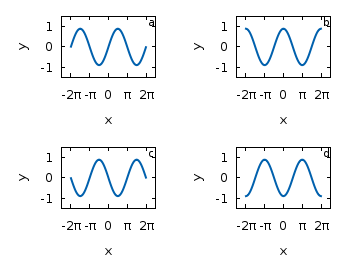Fig. 2 A straightforward use of the multiplot command to plot four different functions (code to produce this figure)

But before we fix this we will introduce the use of macros in order to shorten the code a lot. As you can see in the code block above, the set label command contains the same position for every graph. We can shorten this by:

```set macros
# Placement of the a,b,c,d labels in the graphs
POS = "at graph 0.92,0.9 font ',8'"
[...]
set label 1 'a' @POS
plot f(x) with lines ls 1
```

and so on for the other graphs.

But the macros are not only useful for the different labels, but also for the other settings of the multiplot.
First we want to remove the x/y-tics and labels, where they are not necessary. Here it is the same as with the label settings. Every graph uses the settings from the graph before, if we didn’t change these settings. In order to remove the xtics at a given graph we have to tell this explicitly. Therefore we define macros for the two cases label+tics vs. nolabel+notics:

```# x- and ytics for each row resp. column
NOXTICS = "set xtics ('' -2*pi,'' -pi,'' 0,'' pi,'' 2*pi); \
unset xlabel"
XTICS = "set xtics ('-2π' -2*pi,'-π' -pi,'0' 0,'π' pi,'2π' 2*pi);\
set xlabel 'x'"
NOYTICS = "set format y ''; unset ylabel"
YTICS = "set format y '%.0f'; set ylabel 'y'"
```

These will then be used in the plotting section:

```# --- GRAPH a
@NOXTICS; @YTICS
[...]
# --- GRAPH b
@NOXTICS; @NOYTICS
[...]
# --- GRAPH c
@XTICS; @YTICS
[...]
# --- GRAPH d
@XTICS; @NOYTICS
```

Applying the axes settings will result in Fig. 3.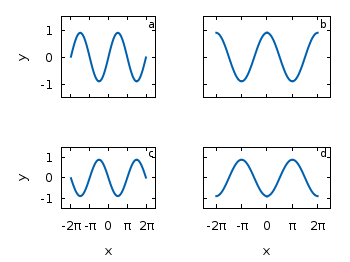Fig. 3 A multiplot with reduced axes labeling (code to produce this figure)

Now the label etc. on the axes are done in a nice way, but the sizes and the spaces between the individual graphs are very bad. This comes from the fact that Gnuplot calculates the size of a graph depending on the presence of tics and labels. In order to have graphs with the same size and align them without spaces between them we have to set the margins of the individual graphs manually.

```# Margins for each row resp. column
TMARGIN = "set tmargin at screen 0.90; set bmargin at screen 0.55"
BMARGIN = "set tmargin at screen 0.55; set bmargin at screen 0.20"
LMARGIN = "set lmargin at screen 0.15; set rmargin at screen 0.55"
RMARGIN = "set lmargin at screen 0.55; set rmargin at screen 0.95"
```

The trick is that we use the `at screen` command to arrange the margins absolutely in the figure. As you can see the bottom margin of the two figures in the top is placed at `0.55`, the same value the top margin of the two lower graphs start.
These margins are then added in the same way to the multiplot command as the label settings and we get:

```# --- GRAPH a
@TMARGIN; @LMARGIN
[...]
# --- GRAPH b
@TMARGIN; @RMARGIN
[...]
# --- GRAPH c
@BMARGIN; @LMARGIN
[...]
# --- GRAPH d
@BMARGIN; @RMARGIN
```

Having done all this we will finally get our desired figure which is shown in Fig. 1.

July 27th, 2010 | 1 Comment

In the introduction I have set the xtics labels manually to use a multiple of π:

```set xtics ('-2π' -2*pi, '-π' -pi, 0, 'π' pi, '2π' 2*pi)
```

But there is an easier way to achieve the same. First we tell Gnuplot to place the tics at multiplies of π. And then the trick: with the `set format` option we can tell Gnuplot to use multiple of π too:

```set xtics pi
set format x '%.0Pπ'
```

In the same way we can place tics without any label by applying:

```set format x ''
```